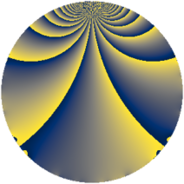# Properties

 Label 3800.1.bnLevel $3800$ Weight $1$ Character orbit 3800.bn Rep. character $\chi_{3800}(1299,\cdot)$ Character field $\Q(\zeta_{6})$ Dimension $12$ Newform subspaces $3$ Sturm bound $600$ Trace bound $11$

# Related objects

## Defining parameters

 Level: $$N$$ $$=$$ $$3800 = 2^{3} \cdot 5^{2} \cdot 19$$ Weight: $$k$$ $$=$$ $$1$$ Character orbit: $$[\chi]$$ $$=$$ 3800.bn (of order $$6$$ and degree $$2$$) Character conductor: $$\operatorname{cond}(\chi)$$ $$=$$ $$760$$ Character field: $$\Q(\zeta_{6})$$ Newform subspaces: $$3$$ Sturm bound: $$600$$ Trace bound: $$11$$

## Dimensions

The following table gives the dimensions of various subspaces of $$M_{1}(3800, [\chi])$$.

Total New Old
Modular forms 44 20 24
Cusp forms 20 12 8
Eisenstein series 24 8 16

The following table gives the dimensions of subspaces with specified projective image type.

$$D_n$$ $$A_4$$ $$S_4$$ $$A_5$$
Dimension 12 0 0 0

## Trace form

 $$12 q + 6 q^{4} + 6 q^{9} + O(q^{10})$$ $$12 q + 6 q^{4} + 6 q^{9} - 6 q^{16} - 6 q^{36} - 12 q^{49} + 6 q^{51} + 12 q^{54} - 6 q^{59} - 12 q^{64} + 6 q^{66} - 6 q^{81} - 12 q^{86} - 6 q^{99} + O(q^{100})$$

## Decomposition of $$S_{1}^{\mathrm{new}}(3800, [\chi])$$ into newform subspaces

Label Dim $A$ Field Image CM RM Traces $q$-expansion
$a_{2}$ $a_{3}$ $a_{5}$ $a_{7}$
3800.1.bn.a $4$ $1.896$ $$\Q(\zeta_{12})$$ $D_{3}$ $$\Q(\sqrt{-2})$$ None $$0$$ $$0$$ $$0$$ $$0$$ $$q-\zeta_{12}^{5}q^{2}+\zeta_{12}^{5}q^{3}-\zeta_{12}^{4}q^{4}+\cdots$$
3800.1.bn.b $4$ $1.896$ $$\Q(\zeta_{12})$$ $D_{3}$ $$\Q(\sqrt{-2})$$ None $$0$$ $$0$$ $$0$$ $$0$$ $$q-\zeta_{12}^{5}q^{2}-\zeta_{12}^{5}q^{3}-\zeta_{12}^{4}q^{4}+\cdots$$
3800.1.bn.c $4$ $1.896$ $$\Q(\zeta_{12})$$ $D_{3}$ $$\Q(\sqrt{-2})$$ None $$0$$ $$0$$ $$0$$ $$0$$ $$q-\zeta_{12}^{5}q^{2}-\zeta_{12}^{5}q^{3}-\zeta_{12}^{4}q^{4}+\cdots$$

## Decomposition of $$S_{1}^{\mathrm{old}}(3800, [\chi])$$ into lower level spaces

$$S_{1}^{\mathrm{old}}(3800, [\chi]) \cong$$ $$S_{1}^{\mathrm{new}}(760, [\chi])$$$$^{\oplus 2}$$